# Python Graphviz 的使用-绘制树形图2021年1月7日07:28:48

2 7749字阅读25分49秒

## 简介

`Graphviz` 是一个绘图工具，可以根据 `dot` 脚本画出树形图等。本文会介绍 `graphviz` 的简单使用，使用 `graphviz` 来创建 `dot` 文件来绘制树形图。本文还会通过几个 `graphviz` 的例子，来介绍 `graphviz` 的使用。

## Graphviz 的简单介绍

### 绘制简单树形图

1. digraph G {
2.     main -> parse -> execute;
3.     main -> init;
4.     main -> cleanup;
5.     execute -> make_string;
6.     execute -> printf;
7.     execute -> compare;
8. }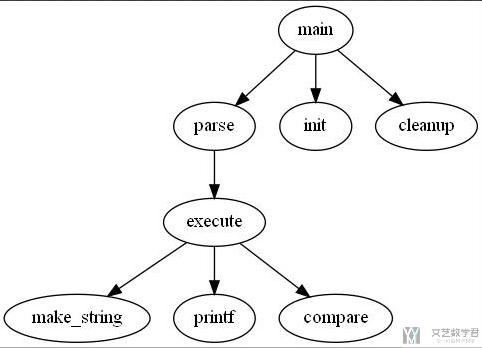### 绘制更加复杂的树形图-node 和 edge 的样式

• 给 node 和 edge 添加格式，颜色，加粗，是否虚线；
• 在箭头上添加文字，例如使用 `a->b [style="dashed", color="skyblue"]`
• 支持中文，在 node 上指定 fontname 即可，`fontname="Microsoft Yahei"`
• 指定箭头的方向，这里在连接部分使用 `dir=none`, `dir=both`, `dir=forward`, `dir=back`

1. digraph G {
2.     main [shape=box];
3.     main -> parse [weight=8];
4.     parse-> execute;
5.     main -> init [style=dotted, dir = none];
6.     main -> cleanup [dir = both];
7.     execute -> {make_string, printf};
8.     init -> make_string;
9.     edge [color=red];
10.     main -> printf [style=bold, label="100 times"];
11.     make_string [label = "make a\nstring"];
12.     node [shape=box, style=filled,color=".7, .3, 1.0", fontname="Microsoft Yahei"];
13.     execute -> 比较;
14. }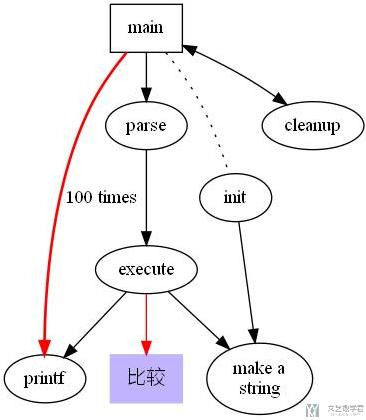### 定义子图和子图的样式

graphviz 支持子图，即图中的部分节点和边相对对立（软件的模块划分经常如此）。比如在下面的例子中，我们将「流量生成」，「批量流量还原」和「真实流量还原」是一个子图。「仿真环境」等是一个模块，完整的代码如下（需要注意，子图的名称必须以 `cluster` 开头，否则 `graphviz` 无法设别）：

1. digraph G {
2.     SUMO_RL [style="filled", fontsize = 20, color="black", fillcolor="chartreuse"];
3.     SUMO_RL -> 流量生成 [color="red"];
4.     subgraph cluster_traffic{
5.         bgcolor="mintcream";
6.         label = "强化学习数据生成";
7.         流量生成 -> 真实流量还原;
8.         流量生成 -> 批量生成流量;
9.     }
10.     强化学习训练 [style="dashed", color="yellowgreen"];
11.     强化学习测试 [style="dashed", color="yellowgreen"];
12.     批量生成流量 -> 强化学习训练 [style="dashed", color="skyblue"];
13.     真实流量还原 -> 强化学习测试 [style="dashed", color="skyblue"];
14.     SUMO_RL -> 仿真环境 [color="red"];
15.     subgraph cluster_rl_env{
16.         bgcolor = "mintcream";
17.         label = "强化学习交互环境";
18.         仿真环境 -> 获得车辆属性;
19.         获得车辆属性 -> 提取环境特征 [label="observation and reward"];
20.         仿真环境 -> 异步控制不同信号灯 [label="action"];
21.     }
22.     与SUMO交互 [style="dashed", color="yellowgreen"];
23.     提取环境特征 -> 与SUMO交互 [style="dashed", color="skyblue"];
24.     异步控制不同信号灯 -> 与SUMO交互 [style="dashed", color="skyblue"];
25. }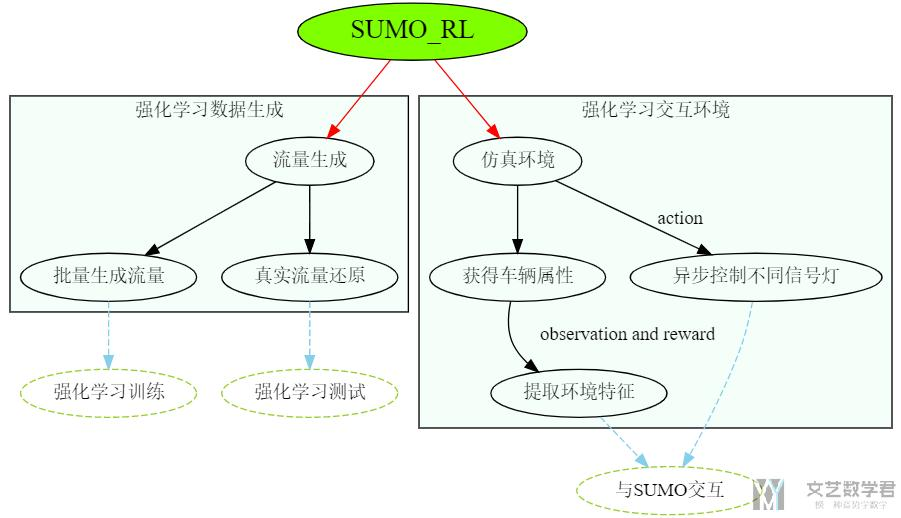### 在 VS Code 中显示 dot 文件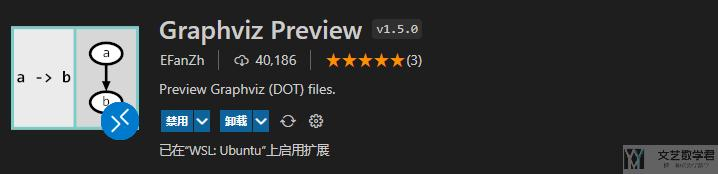## Python Graphviz 简单介绍

1. pip install graphviz

### 最基础的 Python Graphviz 图像

1. from graphviz import Digraph
2. g = Digraph('G', filename='hello.gv')
3. g.node('node1', label='Hello')
4. g.node('node2', label='World')
5. g.edge('node1', 'node2')
6. g.view()
•  上面我们首先初始化了一个 Digraph 类，它可以让我们设置这个 `graph` 的名称，上面是 `'G'`，和最后 `dot` 文件的名称，即 `filename` 这个参数。
• 接着我们使用 `node` 来创建节点，其中第一个参数是 `node``name`，第二个参数是 `node``label`，也就是最终会显示在图中的文字。
• 最后使用 `edge` 来将 `node` 连起来。`edge` 的参数为 `node``name`，第一个为起点，第二个为终点。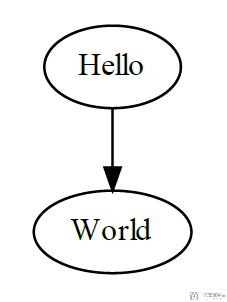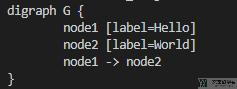### 给树形图增加样式

1. g.edge_attr.update(arrowhead='vee', arrowsize='2') # edge 的样式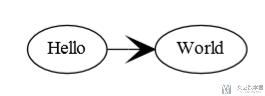1. g.node('node1', label='Hello', shape='star')
2. g.node('node2', label='World', shape='egg')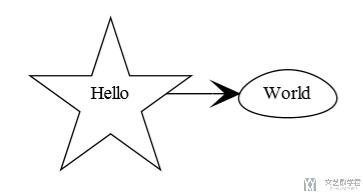1. from graphviz import Digraph
2. g = Digraph('G', node_attr={'shape': 'plaintext'}, filename='hello.gv')
3. g.graph_attr['rankdir'] = 'LR'
4. g.edge_attr.update(arrowhead='vee', arrowsize='2') # edge 的样式
5. g.node('node1', label='Hello', shape='star')
6. g.node('node2', label='World', shape='egg')
7. g.node('tab', label='''<<TABLE>
8.  <TR>
9.    <TD>left</TD>
10.    <TD>right</TD>
11.  </TR>
12. </TABLE>>''')
13. g.edge('node1', 'node2')
14. g.edge('node1', 'tab')
15. g.view()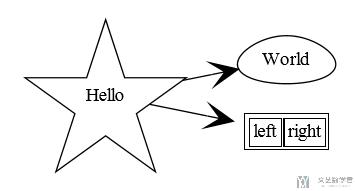## Python Graphviz 例子

### 自定义 node 样式-fsm

1. from graphviz import Digraph
2. f = Digraph('finite_state_machine', filename='fsm.gv')
3. f.attr(rankdir='LR', size='20,5')
4. # 单独定义的 node 会有双圆结构
5. f.attr('node', shape='doublecircle')
6. f.node('LR_0')
7. f.node('LR_3')
8. f.node('LR_4')
9. f.node('LR_8')
10. f.attr('node', shape='circle')
11. f.edge('LR_0', 'LR_2', label='SS(B)')
12. f.edge('LR_0', 'LR_1', label='SS(S)')
13. f.edge('LR_1', 'LR_3', label='S(\$end)')
14. f.edge('LR_2', 'LR_6', label='SS(b)')
15. f.edge('LR_2', 'LR_5', label='SS(a)')
16. f.edge('LR_2', 'LR_4', label='S(A)')
17. f.edge('LR_5', 'LR_7', label='S(b)')
18. f.edge('LR_5', 'LR_5', label='S(a)')
19. f.edge('LR_6', 'LR_6', label='S(b)')
20. f.edge('LR_6', 'LR_5', label='S(a)')
21. f.edge('LR_7', 'LR_8', label='S(b)')
22. f.edge('LR_7', 'LR_5', label='S(a)')
23. f.edge('LR_8', 'LR_6', label='S(b)')
24. f.edge('LR_8', 'LR_5', label='S(a)')
25. f.view()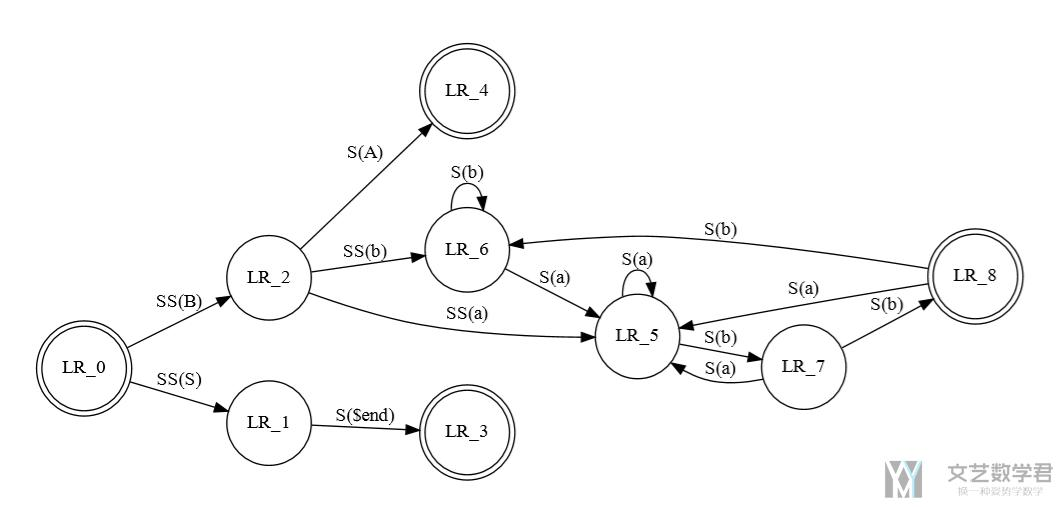### 包含子图的情况

1. from graphviz import Digraph
2. g = Digraph('G', filename='cluster.gv')
3. # NOTE: the subgraph name needs to begin with 'cluster' (all lowercase)
4. #       so that Graphviz recognizes it as a special cluster subgraph
5. with g.subgraph(name='cluster_0') as c:
6.     c.attr(style='filled', color='lightgrey')
7.     c.node_attr.update(style='filled', color='white')
8.     c.edges([('a0', 'a1'), ('a1', 'a2'), ('a2', 'a3')])
9.     c.attr(label='process #1')
10. with g.subgraph(name='cluster_1') as c:
11.     c.attr(color='blue')
12.     c.node_attr['style'] = 'filled'
13.     c.edges([('b0', 'b1'), ('b1', 'b2'), ('b2', 'b3')])
14.     c.attr(label='process #2')
15. g.edge('start', 'a0')
16. g.edge('start', 'b0')
17. g.edge('a1', 'b3')
18. g.edge('b2', 'a3')
19. g.edge('a3', 'a0')
20. g.edge('a3', 'end')
21. g.edge('b3', 'end')
22. g.node('start', shape='Mdiamond')
23. g.node('end', shape='Msquare')
24. g.view()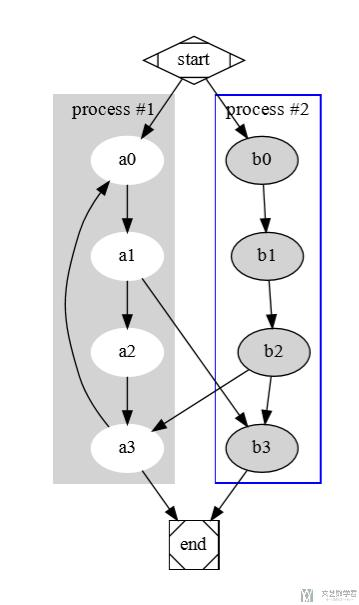### 使用类 html 标签

1. from graphviz import Digraph
2. s = Digraph('structs', filename='structs_revisited.gv',
3.             node_attr={'shape': 'record'})
4. s.node('struct1', '<f0> left|<f1> middle|<f2> right')
5. s.node('struct2', '<f0> one|<f1> two')
6. s.node('struct3', r'hello\nworld |{ b |{c|<here> d|e}| f}| g | h')
7. s.edges([('struct1:f1', 'struct2:f0'), ('struct1:f2', 'struct3:here')])
8. s.view()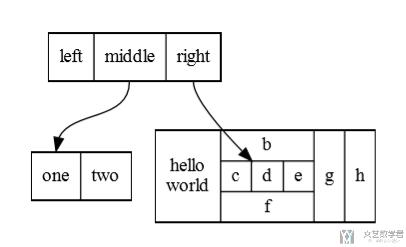1. from graphviz import Digraph
2. s = Digraph('structs', filename='structs_revisited.gv',
3.             node_attr={'shape': 'record'})
4. s.node('struct1', 'left| middle| right')
5. s.node('struct2', '{a|{b1|b2|b3}|c}')
6. s.node('struct3', r'hello\nworld |{ b |{c|d|e}| f}| g | h')
7. s.edges([('struct1', 'struct2'), ('struct1', 'struct3')])
8. s.view()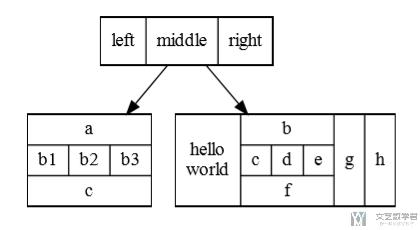### 绘制树结构

1. from graphviz import Digraph, nohtml
2. g = Digraph('g', filename='btree.gv',
3.             node_attr={'shape': 'record', 'height': '.1'})
4. g.node('node0', nohtml('<f0> |<f1> G|<f2>'))
5. g.node('node1', nohtml('<f0> |<f1> E|<f2>'))
6. g.node('node2', nohtml('<f0> |<f1> B|<f2>'))
7. g.node('node3', nohtml('<f0> |<f1> F|<f2>'))
8. g.node('node4', nohtml('<f0> |<f1> R|<f2>'))
9. g.node('node5', nohtml('<f0> |<f1> H|<f2>'))
10. g.node('node6', nohtml('<f0> |<f1> Y|<f2>'))
11. g.node('node7', nohtml('<f0> |<f1> A|<f2>'))
12. g.node('node8', nohtml('<f0> |<f1> C|<f2>'))
13. g.edge('node0:f2', 'node4:f1')
14. g.edge('node0:f0', 'node1:f1')
15. g.edge('node1:f0', 'node2:f1')
16. g.edge('node1:f2', 'node3:f1')
17. g.edge('node2:f2', 'node8:f1')
18. g.edge('node2:f0', 'node7:f1')
19. g.edge('node4:f2', 'node6:f1')
20. g.edge('node4:f0', 'node5:f1')
21. g.view()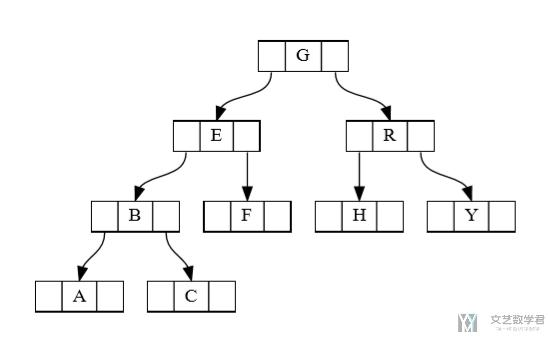• 微信公众号
• 关注微信公众号
•• QQ群
• 我们的QQ群号
•• 本文由 发表于 2021年1月7日07:28:48
• 转载请务必保留本文链接：https://mathpretty.com/13259.html